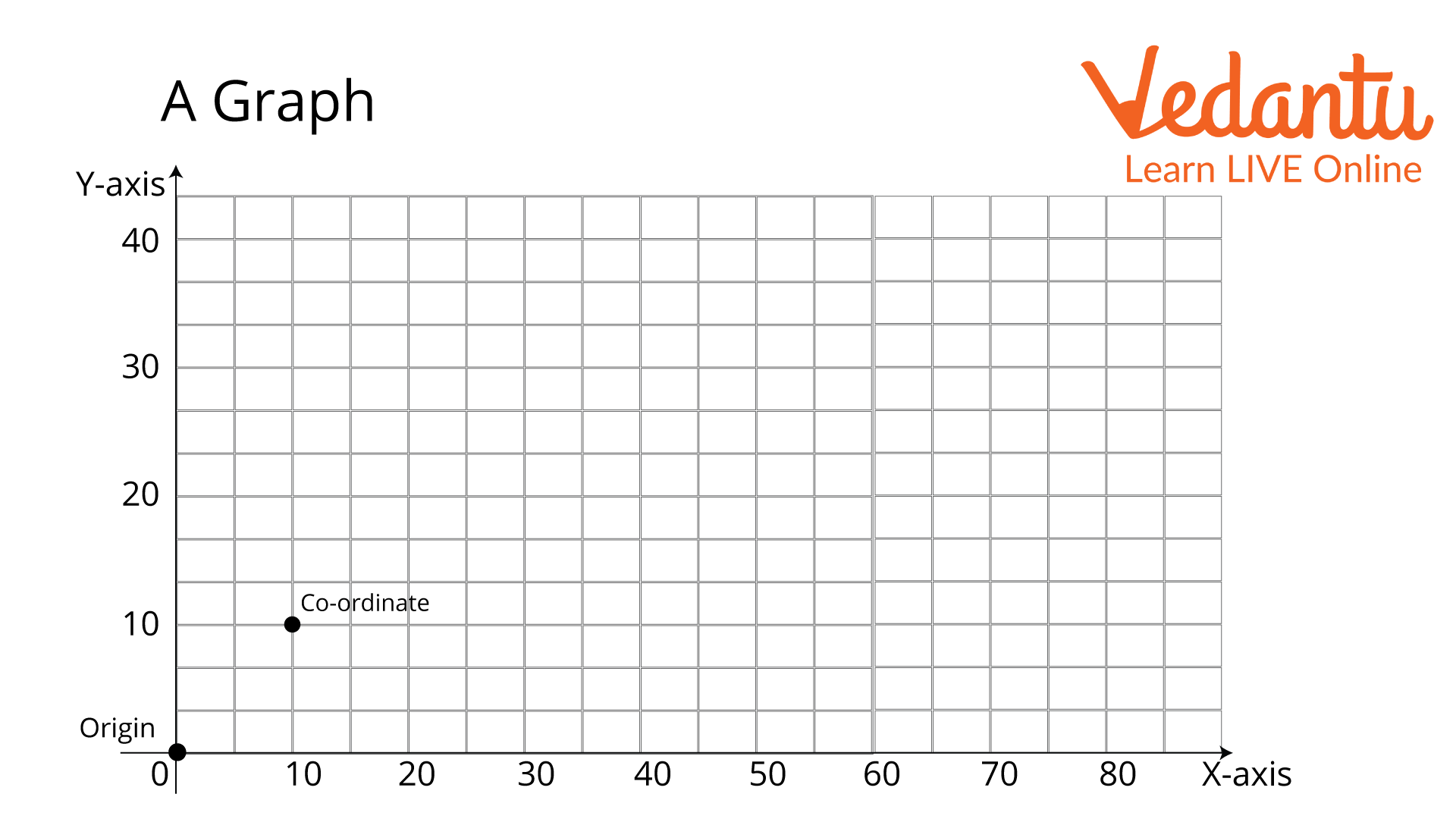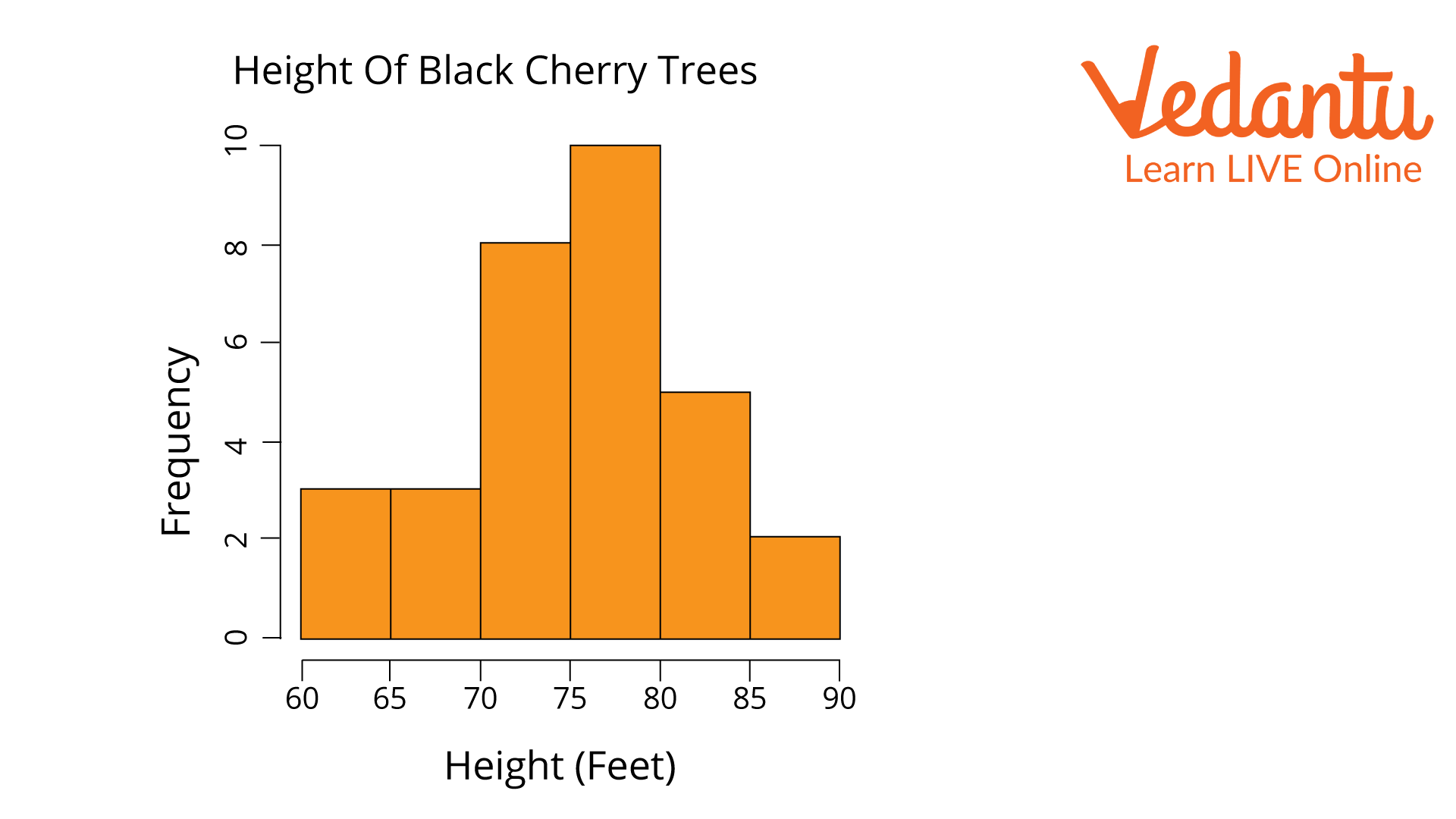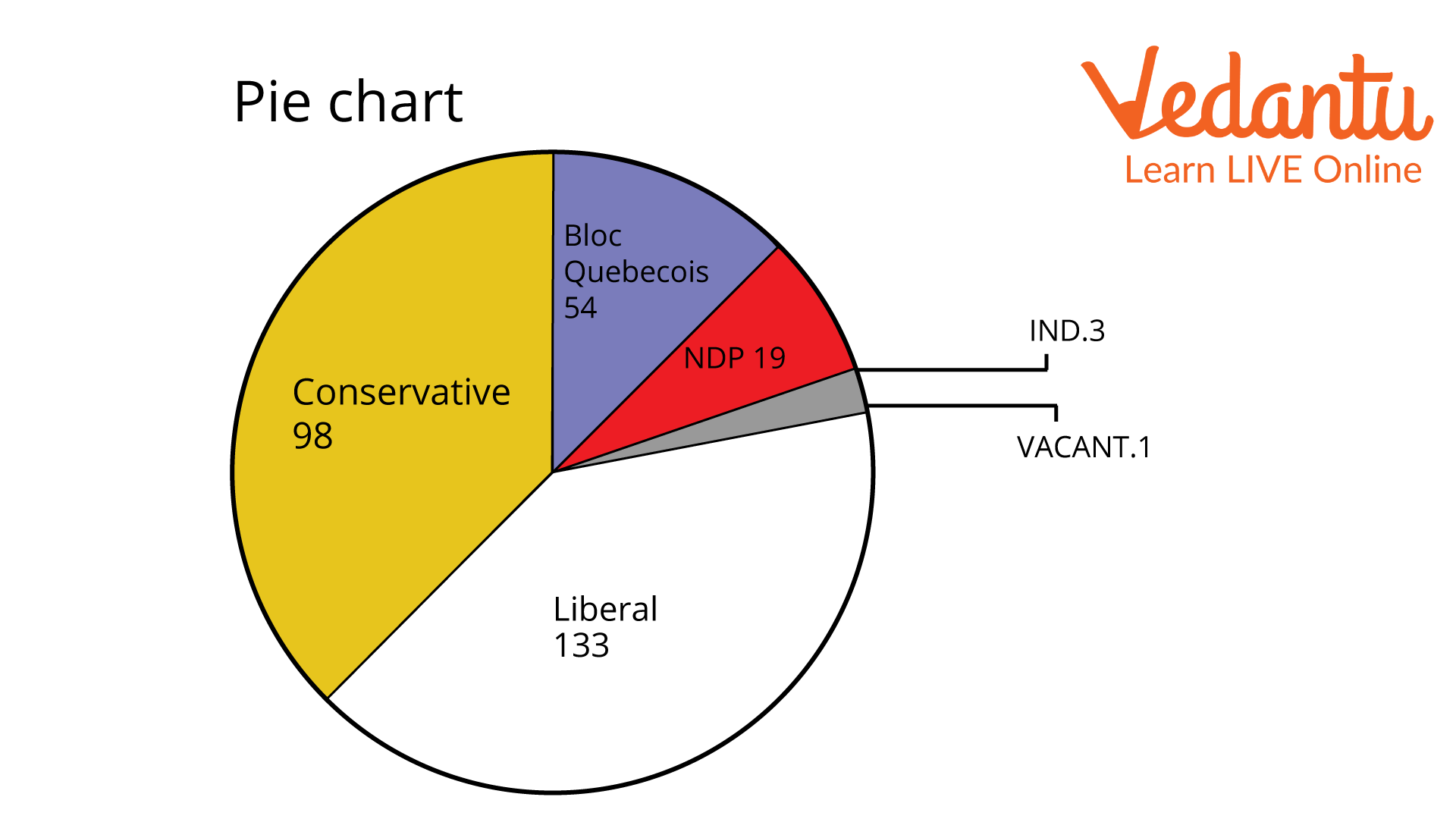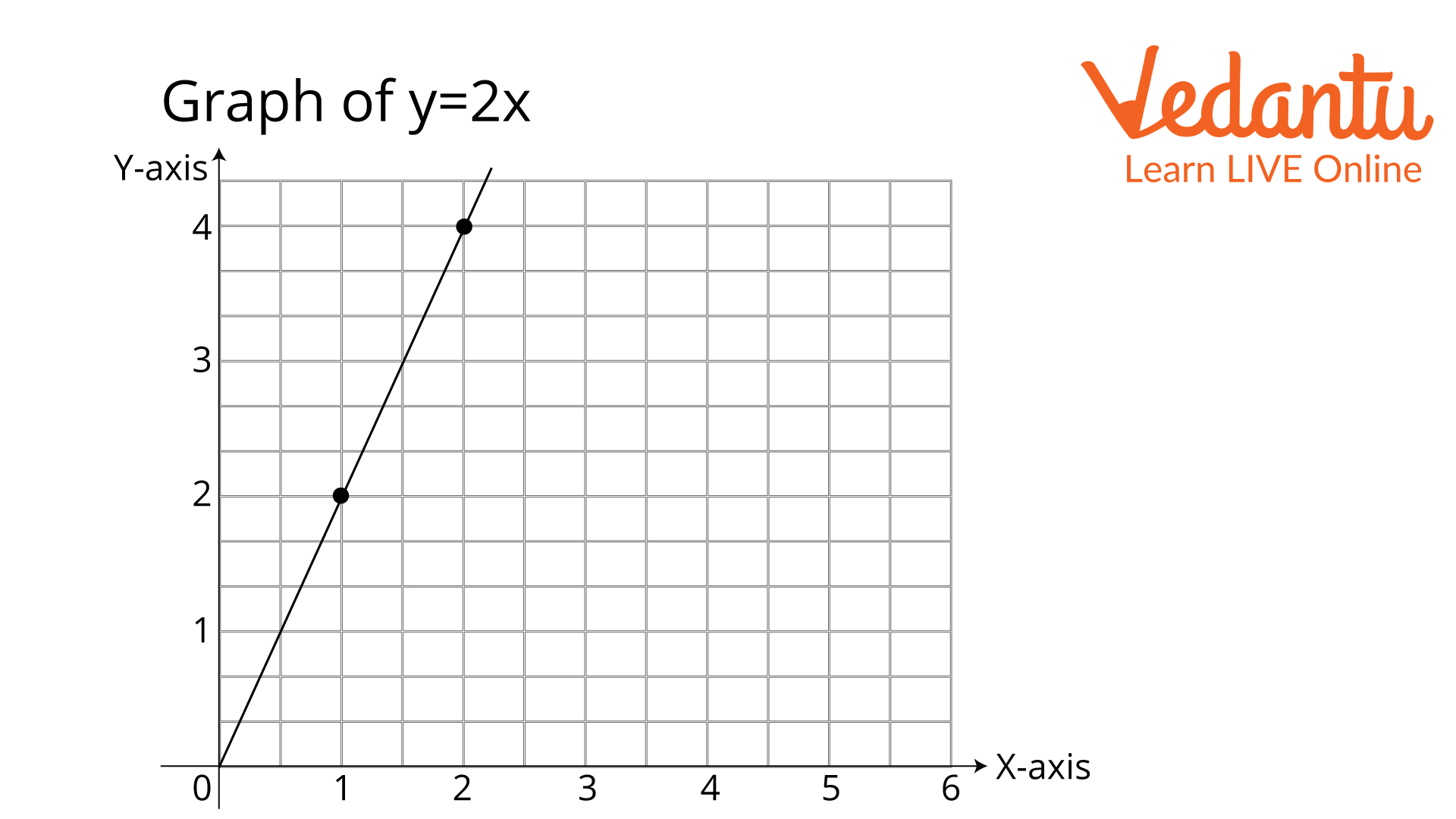Courses
Courses for Kids
Free study material
Offline Centres
More

# Drawing a GraphLast updated date: 03rd Dec 2023
Total views: 99.6k
Views today: 2.99k## Introduction to Graphs

A graph is a diagram that shows the relationship between two different quantities. Varying quantities are the quantities that change with respect to other conditions. In a graph, these varying quantities are represented on two lines called axes. The two axes form a right angle with each other. The two axes are called the X-axis and the Y-axis. Another name for the X-axis is abscissa, and the Y-axis is ordinate.

Now that you have understood the graph and the axes let us move forward to drawing a graph.

## A Graph and Its FunctionsA Graph

You can observe from the above figure that the horizontal line is labelled as the X-axis or the abscissa, and the vertical line has been labelled as the Y-axis or the ordinate. You can also observe that both are making a right angle with each other. The point where these axes intersect is known as the origin, marked at zero, as shown in the image above. A graph is formed when many coordinates are connected to each other.

## Types of Graphs

There are many types of graphs, like bar graphs, column graphs, histograms, frequency polygons, and many more; however, you will need to learn four basic types, which are given below:

• Line Graph

In this type of graph, only coordinates are plotted on the graph, and these coordinates are connected together to form a line that is sometimes straight or curved. We will learn to draw a line graph later in this article.

• Bar Graph

A bar graph is a chart or the visual representation of any quantity with the help of bars or strips. These bars or strips look like columns.

As shown below, a histogram is a bar graph that depicts frequency distribution.Histogram

• ## Pie Chart

It is a circular figure that is divided into slices to show the proportion of the given quantities. Given below is a representation of a pie chart.Pie chart

## Steps to Draw a Graph

The line graph is the simplest form of a graph. Therefore, before proceeding to draw a graph of various types, you must learn to draw a line graph first. Therefore, let us first start by drawing a graph of x and y coordinates. Let us take an example so that you can understand the steps one by one. The question says, “Draw a graph with the variable y being dependent on the variable x by the relation y = 2x.” Here are the steps based on the example:

1. Determine the Given Variables

Since the instruction is to “Draw a graph with the variable y being dependent on the variable x by the relation y=2x.” This means that for any value of x, the value of y will be doubled.

1. Form a Table of X and Y Coordinates

Since you have determined the relationship between the variables, you can form a table of x and y coordinates as shown below.

Table of coordinates

 x 0 1 2 y = 2x 0 2 4

1. Plot the Point

After you form a table and arrange the given data, plot the points on graph paper.

Take the scale of a graph as 1 unit = 10mm for x and 1 unit = 10mm for y. Start with the x coordinate and mark the point for the corresponding value given in the table.## Conclusion

This is how you make a line graph. Wasn't it interesting to learn something new about data organisation? So, in this article, you learnt about graphs, graph types, and the two axes. You have also learnt the simple steps for drawing graphs with various data, which are to determine the variables, draw a table of the arranged data, and then draw the diagram. You have also learnt to take the scale of the graph into account when drawing one.

## FAQs on Drawing a Graph

1. What are the 3 essential parts of a graph?

The 3 most essential parts of a graph are the two axes, the scaling of the graph, and the data. The data must be provided in the question for the graph to be valid. The data can be in the form of a sentence, which you can write in the form of a table. While drawing the graph, you must also mark the axes as X and Y-axes to avoid confusion. The scale is also an essential part of a graph; for example, 1 unit equals 10 mm.

2. What are the types of questions that we can solve with the help of graphs?

The types of questions that we can solve with the help of graphs are function-related questions and many more. As you reach higher classes, you will use graphs to solve way more complex questions like linear equations, etc.

3. What are functions and what is their relationship with a graph?

A function is a relationship that involves more than one variable. For example, in the question we solved above, y = 2x is also a function. These kinds of functions where more than one variable is involved are solved using graphs, especially linear graphs. This is the relationship between function and graphs.